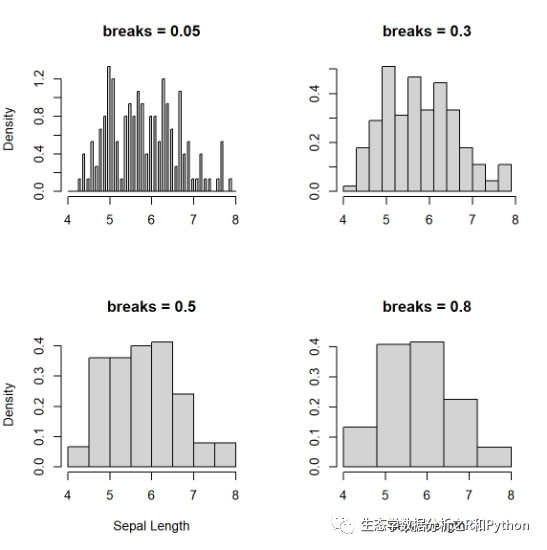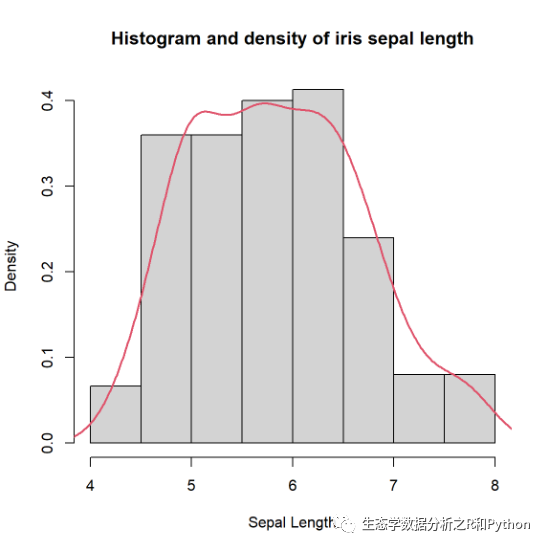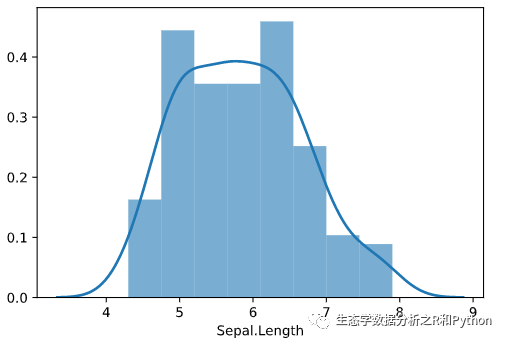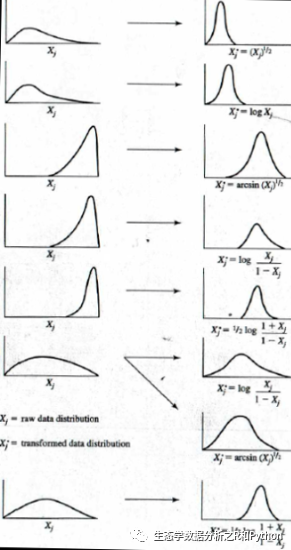• 肉眼判断

• 假设检验

# 肉眼判断

## 直方图和密度曲线的比较

layout(matrix(c(1, 2, 3, 4), 2, 2, byrow = TRUE))hist(  iris$Sepal.Length, freq = FALSE, breaks = c(seq(4, 8, 0.05)), main = "breaks = 0.05", xlab = NULL)hist( iris$Sepal.Length,  freq = FALSE,  breaks = c(seq(4, 8, 0.3)),  main = "breaks = 0.3",  xlab = NULL,  ylab = NULL)hist(  iris$Sepal.Length, freq = FALSE, breaks = c(seq(4, 8, 0.5)), main = "breaks = 0.5", xlab = "Sepal Length")hist( iris$Sepal.Length,  freq = FALSE,  breaks = c(seq(4, 8, 0.8)),  main = "breaks = 0.8",  xlab = "Sepal Length",  ylab = NULL)hist(  iris$Sepal.Length, freq = FALSE, xlab = "Sepal Length", main = "Histogram and density of iris sepal length")lines(density(iris$Sepal.Length), col = 2, lwd = 2)python 版本的验证：

import pandas as pdimport statsmodels.api as smimport matplotlib.pyplot as pltimport seaborn as snsiris = pd.read_csv("./data/iris.csv")kwargs = dict(hist_kws={'alpha':.6}, kde_kws={'linewidth':2})plt.figure(figsize=(10,7), dpi= 80)ax = sns.distplot(iris["Sepal.Length"], label="Sepal Length histogram and density", **kwargs)ax.set_xlim([4,8])####  Shapiro-Wilk normality test#### data:  iris$Sepal.Length## W = 0.97609, p-value = 0.01018 非常简单，一行代码搞定，结果让我们非常失望，数据不是正态分布。事实证明，上面的肉眼判断，我的看法是错误的。 python 的 scipy 中也有同样的方法： from scipy import statsimport pandas as pdiris = pd.read_csv("./data/iris.csv")stats.shapiro(iris["Sepal.Length"]) ## (0.9760899543762207, 0.010180278681218624) 尽管值略有差别，充分体现了主观的判断的不靠谱。那我们只能试试怎么将数据转换为正态分布的方法了。 # 常用的正态转换方法 当然，如果样本量超过了30，或者50更好，正态分布的检验并非十分重要。当然转换为正态分布的方法比较多，下面的一幅图即可概括：来自 Applied Multivariate Statistics for the Social Sciences 方法这么多，其实也没太多特别的，就是将数据转换一下，例如我们使用最简单的对数转换看一下： shapiro.test(log10(iris$Sepal.Length))
####  Shapiro-Wilk normality test#### data:  log10(iris\$Sepal.Length)## W = 0.98253, p-value = 0.05388

# 参考资料

Amrhein, Valentin, Sander Greenland, and Blake McShane. 2019. “Scientists Rise up Against Statistical Significance.” Nature. https://doi.org/10.1038/d41586-019-00857-9.

DeepAI. 2017. “What Is a Kernel Density Estimation?” https://deepai.org/machine-learning-glossary-and-terms/kernel-density-estimation.

Ed. 2017. “Transforming Data for Normality.” https://www.statisticssolutions.com/transforming-data-for-normality/.

Ford, Clay. 2017. “Understanding Q-Q Plots.” https://data.library.virginia.edu/understanding-q-q-plots/.

“Kernel Density Estimation(KDE).” 2017. https://blog.csdn.net/unixtch/article/details/78556499.

R Core Team. 2020. R: A Language and Environment for Statistical Computing. Vienna, Austria: R Foundation for Statistical Computing. https://www.R-project.org/.

Wasserstein, Ronald L., Allen L. Schirm, and Nicole A. Lazar. 2019. “Moving to a World Beyond ‘P < 0.05’.” The American Statistician. https://doi.org/10.1080/00031305.2019.1583913.

12-03146009-261万+
01-01395
11-2723
11-2935
12-23569
06-293万+
03-062904
01-111272
01-12885
02-21662
02-122194
05-311万+
12-11668
11-23244
08-131022
12-091万+点击重新获取扫码支付余额充值